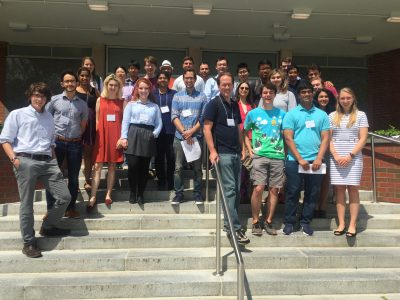# Geodesic Interpolation on the Sierpinski Gasket

July 9, 2018

## Overview

Geodesics (shortest paths) on manifolds such as planes and spheres are well understood.  Geodesics on fractal sets such as the Sierpinski Triangle are much more complicated.  We begin by constructing algorithms for building shortest paths and provide explicit formulas for computing their lengths.  We then turn to the question of interpolation along geodesics—given two subsets of the Sierpinski Triangle, we “slide” points in one set along geodesics to the other set.  We construct a measure along the interpolated sets which formalizes a notion of the interpolation of a distribution of mass, and we prove interesting self-similarity relations about this measure.

# 5th Northeast Mathematics Undergraduate Research Mini-Symposium

August 17, 2017

### University of Connecticut, August 3rd, 2017

#### Participating Schools: Amherst, Smith, UConn and UMass

5th Mini-Symposium full program (2017)July 28, 2017

## Overview

Laplacians have been well studied on post-critically finite (PCF) fractals. However, less is known about gradients on such fractals. Building on work by Teplyaev, we generalize results regarding the existence and continuity of the gradient on the standard Sierpinski Gasket to higher dimensional Sierpinski Gaskets. In particular, we find that, for functions with a continuous Laplacian, the gradient must be defined almost everywhere, and specify a set of points for which it is defined. Furthermore, we provide a counterexample on higher-dimensional Sierpinski gaskets where the Laplacian is continuous but the gradient is not defined everywhere. We conjecture that Hölder continuity of the Laplacian is a condition strong enough to guarantee that the gradient exists at each point.

# Spectrum of the Magnetic Laplacian on the Diamond Fractal

May 22, 2016

## Overview

The diamond fractal is a fractal that is obtained in the following manner.  Start with a graph with two vertices and an edge and replace the edge with two new vertices connected to our original vertices to obtain a diamond shaped graph.   The diamond fractal is defined to be the limiting object after continuing with the edge replacement indefinitely.  In the project, the spectrum of magnetic Laplacian operators on graph approximations to the diamond fractal was computed.

Given a level n approximation to the fractal with known magnetic field strengths through cells and holes, it is possible to determine the net magnetic field through the cells and holes of the preceding graph approximations.  The spectral similarity relation between the operators on successive graph approximations was worked out, with the corresponding spectral decimation polynomial depending on the magnetic field strengths.  A poster and talk on this work was presented at the REU Mini-Symposium at UConn.

# arXiv:1704.01609

## Presentation

Magnetic Spectral Decimation

Poster